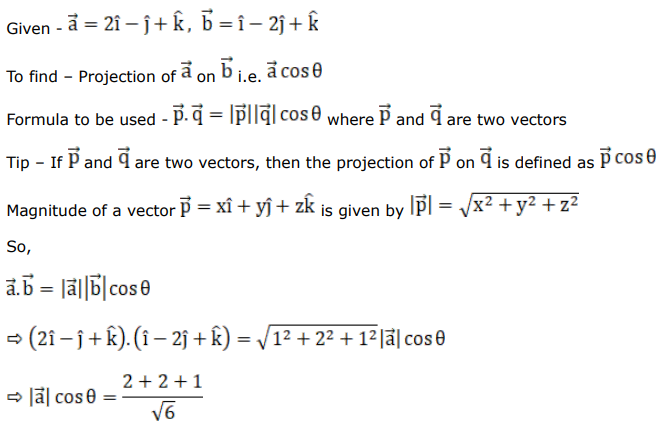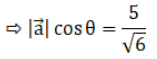# Mark against the correct answer in each of the following:Question:

Mark $(\sqrt{)}$ against the correct answer in each of the following:

What is the projection of $\vec{a}=(2 \hat{j}-\hat{j}+\hat{k})$ on $\overrightarrow{\mathrm{b}}=(\hat{\mathrm{i}}-2 \hat{\mathrm{j}}+\hat{\mathrm{k}})$ $?$

A. $\frac{2}{\sqrt{3}}$

B. $\frac{4}{\sqrt{5}}$

C. $\frac{5}{\sqrt{6}}$

D. none of these

Solution: